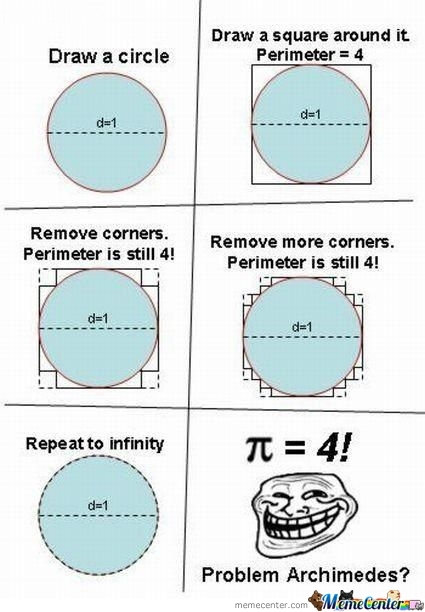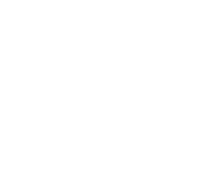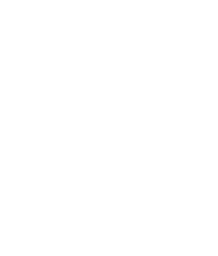# The mystery of π = 4

π must be the most famous of the numbers… people seem to make several strange associations as to its meaning. There is even a sci-fi movie in which they can discover the last decimal digit of π (although this hypothetical “digit” does not exist), and this allows the protagonist to predict variations in stock exchanges around the world. Despite all this popularity, the meaning of π is a little “more boring” than it looks. π means the perimeter of a circle of diameter 1 unit.However, the perimeter of this circle is an Irrational Number, so it can only be treated in decimal calculations by its approximation. Although some people find it legal to remember many decimal places of the approximation of π, honestly for any problem that you need an approximation of better than 3,141592 you will probably be solving with some software, which will give you about 100 places of the π automatically.

In this text we discuss about a meme that circulates involving the “calculation” of π so that it gives 4.In some responses to this meme, those who criticize it suggest that the figure formed should be a rhombus … but this conclusion is wrong. We can actually have a “circle” of diameter 1 and perimeter 4. But to understand how it is possible to build a “circle” in these conditions, it is interesting to first discuss a little bit about fractals.

Imagine an equilateral triangle on side 1. Without any mystery, we know that its perimeter is equal to 3.So we segment each of its edges into three parts. And in the center of each edge, we create a new equilateral triangle with a 1/3 side. Now this new figure has 12 edges, each 1/3 size, so its perimeter is 4.So we segment each of its edges into three parts. And in the center of each edge, we create a new equilateral triangle with a 1/9 side. Now this new figure has 48 edges, each 1/9 size, so its perimeter is approximately 5,33.So we segment each of its edges into three parts. And in the center of each edge, we create a new equilateral triangle with side 1/27. Now this new figure has 192 edges, each 1/27 in size, so its perimeter is approximately 7,11.So we segment each of its edges into three parts. And in the center of each edge, we create a new equilateral triangle with side 1/81. Now this new figure has 768 edges, each 1/81 in size, so its perimeter is approximately 9,48.The process can be continued indefinitely. Taking the construction of a figure known as Koch Island, which has a finite area, but infinite perimeter. A peculiar characteristic of this figure is that its entire perimeter is formed by inflection points (nozzles), which makes it non-derivable for any point.

This same principle can be applied to transform a square into a “circle”. Transforming each vertex with an internal angle of 90 degrees, in other three vertices as shown in the meme, we preserve the perimeter of the figure, however we force that its area is equal to the area of ​​a circle.

So, no matter how close we get, the figure will always look like a circle (because the process has been repeated infinitely), however like the case of Koch Island, this “circle” is all formed by inflection points (nozzles), which makes it non-derivable for any point (detail: circles are derivable at all points).

### 2 thoughts on “The mystery of π = 4”

•12 de maio de 2022 em 14:36
•